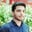Related Tags

loops
objective c
communitycreator

# What are loops in Objective-C?Salman Yousaf

### Overview

What do we do if we want to execute a set of instructions for a specific time or till the condition fails or persists? Here, the loops concept comes to repeat instructions for specific times. Objective-C provides us with the following loops that help us implement our logic.

## Why loops?

Loops provide great assistance to the programmers, because they are used to create a piece of code that can be used repeatedly. In addition, loops are also used to minimize the number of lines in the code. At the same time, they reduce the complexity of the code.

Let's look at the different types of loops available in Objective-C.

### 1. The for Loop

The for loop is used when you want to execute a particular statement for specific iterations—also known as a controlled iterations loop.

### Syntax

for(int variable; condition; increment) {
//body
}

### Example

In the code snippet below, we are going to use for loop to execute instructions in its body 10 times.

#import <Foundation/Foundation.h>
int main () {
NSAutoreleasePool * pool = [[NSAutoreleasePool alloc] init];
int i;
for(i= 0; i <= 10; i++) {
NSLog(@"value of i: %d\n", i);
}
[pool drain];
return 0;
}
for loop
• Line 4: We declare an integer variable, i, that will be used inside the for loop for holding and displaying the values.
• Line 5: We define the for loop with a starting value, i=0, conditional value, i is less than or equal to 10, and incremental value i is equal to the current value plus 1.
• Line 6: We print the resultant value after each iteration until the loop ends.

### 2. The while Loop

The while loop is used to repeat the execution of a set of statements until the condition is true. The loop only ends when the given condition is false.

### Syntax

while(condtiton) {
// body
}

### Example

In the code snippet below, we use a while loop with an initial value k=10 that terminates at k=40.

#import <Foundation/Foundation.h>
int main () {
NSAutoreleasePool * pool = [[NSAutoreleasePool alloc] init];
int k = 10;
while(k <=40) {
NSLog(@"value of k: %d\n", k);
k=k+2;
}
[pool drain];
return 0;
}
while loop
• Line 4: We declare and assign an initial value, 10, to the integer variable k.
• Line 5: We use a while loop and place a condition in it, which says that repeat until k is less than or equal to 40.
• Line 6: We print the value stored in the k. The loop will start by displaying k=10.
• Line 7: After displaying the value of k, we add 2 in the current value of k. This will be the new value of k that would be checked.

### 3. The do-while Loop

The do-while loop is used to execute the statement comparing it with the conditions, which means that do executes the statements for once whether a condition is false or true.

### Syntax

do {
// Statements to execute;
} while(condition);

### Example

In the code snippet below, we are going to use the do-while loop.

#import <Foundation/Foundation.h>
int main () {
NSAutoreleasePool * pool = [[NSAutoreleasePool alloc] init];
int h = 40;
do {
h = h + 2;
NSLog(@"value of h: %d\n", h);
} while( h < 40 );
[pool drain];
return 0;
}
do-while loop
• Line 4: We declare and initialize the integer variable h=40.
• Line 5: We open a do-while loop.
• Line 6:  We add 2 in the current value of h. This will make h=42.
• Line 7:  We print the value of h.
• Line 8:  We check the condition if the value of h is less than 40. Next, we shift the control to the loop else terminate the loop.

RELATED TAGS

loops
objective c
communitycreator

CONTRIBUTORSalman Yousaf
RELATED COURSES

View all Courses

Keep Exploring

Learn in-demand tech skills in half the time